Next: Conserved Current and Charge Up: Klein-Gordon Equation Previous: Solutions of the Klein-Gordon

# General Solution of the Klein-Gordon Equation

Besides plane-wave solutions of definite momentum, we can have solutions which are the Fourier transform of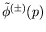which depend only on momentum: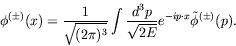(4.23)

The relativistic invariance of solutions of this form is not immediately obvious.

The general solution to the Klein-Gordon equation has either positive or negative energy. They can be written (using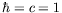) as a Fourier transform of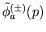,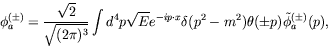(4.24)

where the index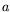is used to label different solutions of identical mass. This form is manifestly Lorentz invariant. The Lorentz invariant step function is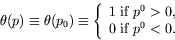(4.25)

Lorentz invariance of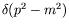restricts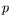to be a time-like vector and thus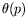distinguishes between past and future. Thus the expression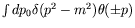ensures the condition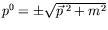.

We can show that equation 4.24 gives rise to the usual form of the solutions by rewriting the general solution, using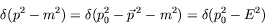(4.26)

and then applying the identity equation 2.28.

For positive energy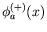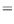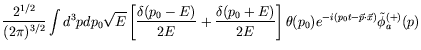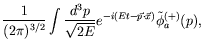(4.27)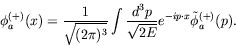(4.28)

For negative energy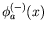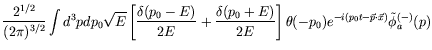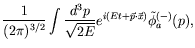(4.29)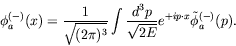(4.30)

Equations 4.28 and 4.30 are identical to the previously solutions (equation 4.23).Next: Conserved Current and Charge Up: Klein-Gordon Equation Previous: Solutions of the Klein-Gordon
Douglas M. Gingrich (gingrich@ ualberta.ca)
2004-03-18